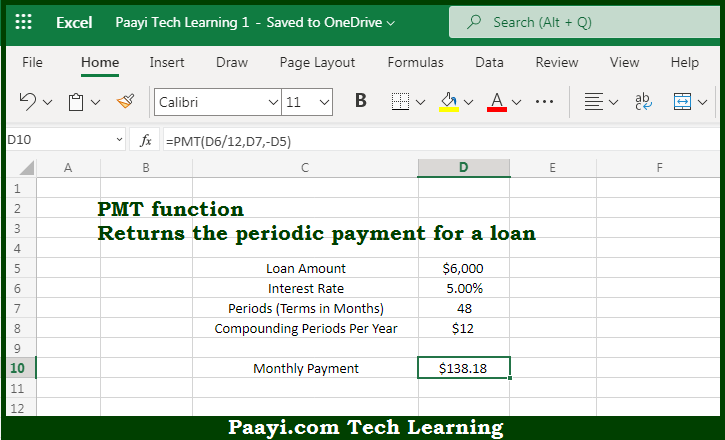# Learn How to Use Microsoft Excel PMT Function

Written by | 0 Comments | 546 Views

In this article, you will learn how to use the Microsoft Excel PMT function and its prime function in Microsoft Excel. You will also get to know the Microsoft Excel PMT function return value and syntax with the help of some examples.

Microsoft Excel PMT Function

The main purpose of the Microsoft Excel PMT function is to get the period payment for the loan. That implies, with the help of the PMT function you can able to return the periodic payment for the loan. The PMT function can be used to figure out payments for a loan, when the loan amount, number of periods, and interest rate are given. So, with the help of the PMT function, you can able to get the period payment for the loan.

Return Value of PMT Function

The return value will be the loan payment as a number.

Syntax of PMT Function

=PMT(rate, nper, pv, [fv], [type])

Where the arguments:

• rate - This is the interest rate of the loan.
• nper: This is the total number of payments for the loan.
• pv: This is the present value or total value of all loan repayments now.
• fv: This is the future value or a cash balance you required after the last payment is made and Default is 0 (optional).
• type: In case the payments are due, then 0 = end of the period and 1 = beginning of the period. Default is 0 (optional).

How to Use Microsoft Excel PMT Function?So we know that Microsoft Excel PMT function you can able to get the period payment for the loan. That implies, with the help of the PMT function you can able to return the periodic payment for the loan. The PMT function can be used to figure out payments for a loan, when the loan amount, number of periods, and interest rate are given. So, with the help of the PMT function, you can able to get the period payment for the loan.